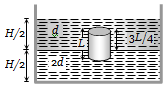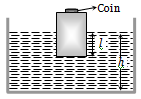Water flows in a streamlined manner through a capillary tube of radius a, the pressure difference being P and the rate of flow Q. If the radius is reduced to a/2 and the pressure increased to 2P, the rate of flow becomes

(a) $4\mathrm{Q}$                          (b) $\mathrm{Q}$

(c) $\frac{\mathrm{Q}}{4}$                          (d) $\frac{\mathrm{Q}}{8}$

Concept Questions :-

Viscosity
High Yielding Test Series + Question Bank - NEET 2020

Difficulty Level:

Water is flowing in a pipe of diameter 4 cm with a velocity 3 m/s. The water then enters into a tube of diameter 2 cm. The velocity of water in the other pipe is
(a) 3 m/s                                        (b) 6 m/s
(c) 12 m/s                                       (d) 8 m/s

Concept Questions :-

Equation of continuity
High Yielding Test Series + Question Bank - NEET 2020

Difficulty Level:

A manometer connected to a closed tap reads $4.5×{10}^{5}$ pascal. When the tap is opened the reading of the manometer falls to $4×{10}^{5}$ pascal. Then the velocity of flow of water is
1. 7  ${\mathrm{ms}}^{-1}$                             2. 8 ${\mathrm{ms}}^{-1}$
3. 9 ${\mathrm{ms}}^{-1}$                              4. 10 ${\mathrm{ms}}^{-1}$

Concept Questions :-

Bernoulli theorem
High Yielding Test Series + Question Bank - NEET 2020

Difficulty Level:

What is the velocity v of a metallic ball of radius r falling in a tank of liquid at the instant when its acceleration is one-half that of a freely falling body ? (The densities of metal and of liquid are $\mathrm{\rho }$ and $\mathrm{\sigma }$ respectively, and the viscosity of the liquid is $\mathrm{\eta }$).

(a) $\frac{{\mathrm{r}}^{2}\mathrm{g}}{9\mathrm{\eta }}\left(\mathrm{\rho }-2\mathrm{\sigma }\right)$                     (b) $\frac{{\mathrm{r}}^{2}\mathrm{g}}{9\mathrm{\eta }}\left(2\mathrm{\rho }-\mathrm{\sigma }\right)$

(c) $\frac{{\mathrm{r}}^{2}\mathrm{g}}{9\mathrm{\eta }}\left(\mathrm{\rho }-\mathrm{\sigma }\right)$                        (d) $\frac{2{\mathrm{r}}^{2}\mathrm{g}}{9\mathrm{\eta }}\left(\mathrm{\rho }-\mathrm{\sigma }\right)$

Concept Questions :-

Viscosity
High Yielding Test Series + Question Bank - NEET 2020

Difficulty Level:

Consider the following equation of Bernoulli’s theorem-

$\mathrm{P}+\frac{1}{2}{\mathrm{\rho V}}^{2}+\mathrm{\rho gh}=\mathrm{K}\left(\mathrm{constant}\right)$

The dimensions of K/P are the same as that of which of the following?

(a) Thrust                         (b) Pressure
(c) Angle                          (d) Viscosity

Concept Questions :-

Bernoulli theorem
High Yielding Test Series + Question Bank - NEET 2020

Difficulty Level:

An incompressible fluid flows steadily through a cylindrical pipe which has radius 2r at point A and radius r at B further along the flow direction. If the velocity at point A is v, its velocity at point B is

(a) 2v                                (b) v
(c) v/2                               (d) 4v

Concept Questions :-

Equation of continuity
High Yielding Test Series + Question Bank - NEET 2020

Difficulty Level:

A homogeneous solid cylinder of length L(L<H/2) . Cross-sectional area A/5 is immersed such that it floats with its axis vertical at the liquid-liquid interface with length L/4 in the denser liquid as shown in the fig. The lower density liquid is open to atmosphere having pressure ${\mathrm{P}}_{0}$. Then density D of solid is given by(a) $\frac{5}{4}\mathrm{d}$                      (b) $\frac{4}{5}\mathrm{d}$

(c) $\mathrm{d}$                          (d) $\frac{d}{5}$

Concept Questions :-

Archimedes principle
High Yielding Test Series + Question Bank - NEET 2020

Difficulty Level:

A wooden block, with a coin placed on its top, floats in water as shown in fig. The distance l and h are shown there. After some time the coin falls into the water. Then(a) l decreases and h increases

(b) l increases and h decreases

(c) Both l and h increase

(d) Both l and h decrease

Concept Questions :-

Archimedes principle
High Yielding Test Series + Question Bank - NEET 2020

Difficulty Level:

A block of ice floats on a liquid of density 1.2 $g/c{m}^{3}$ in a beaker. Then level of liquid when ice completely melts-

(a) Remains same                         (b) Rises
(c) Lowers                                    (d) (a), (b) or (c)

Concept Questions :-

Archimedes principle
High Yielding Test Series + Question Bank - NEET 2020

Difficulty Level:

A vessel of area of cross-section A has liquid to a height H. There is a hole at the bottom of vessel having area of cross-section a. The time taken to decrease the level from ${\mathrm{H}}_{1}$ to ${\mathrm{H}}_{2}$ will be

(a)$\frac{\mathrm{A}}{\mathrm{a}}\sqrt{\frac{2}{\mathrm{g}}}\left[\sqrt{{\mathrm{H}}_{1}}-\sqrt{{\mathrm{H}}_{2}}\right]$              (b) $\sqrt{2\mathrm{gh}}$

(c) $\sqrt{2\mathrm{gh}\left({\mathrm{H}}_{1}-{\mathrm{H}}_{2}\right)}$                      (d) $\frac{\mathrm{A}}{\mathrm{a}}\sqrt{\frac{g}{2}}\left[\sqrt{{\mathrm{H}}_{1}}-\sqrt{{\mathrm{H}}_{2}}\right]$

Concept Questions :-

Bernoulli theorem